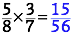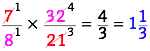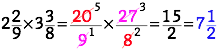# Reducing, Reusing, Recycling

## OBJECTIVE: To learn how to multiply fractions and mixed numbers

In previous lessons, you learned how to add and subtract FRACTIONS and MIXED NUMBERS. Wearing Uniforms: https://www.geogebra.org/m/ceyu3wpa Mixing 'Em Up: https://www.geogebra.org/m/hzx95nwa In this lesson, you'll learn how to multiply FRACTIONS and MIXED NUMBERS. Multiplying FRACTIONS involves three steps (analogous to waste management). However, steps 1 and 3 are sometimes unnecessary. 1. REDUCE: Cancel common factors, if any, from the numerator and the denominator. 2. REUSE: Multiply the reduced numbers, numerator to numerator and denominator to denominator. 3. RECYCLE: Change any improper fractions to mixed numbers. Examples:Multiplying MIXED NUMBERS involves the same three steps as above, but a preliminary step must be carried out—change any mixed numbers to improper fractions before proceeding.Below is a set of problems involving multiplying fractions and mixed numbers.